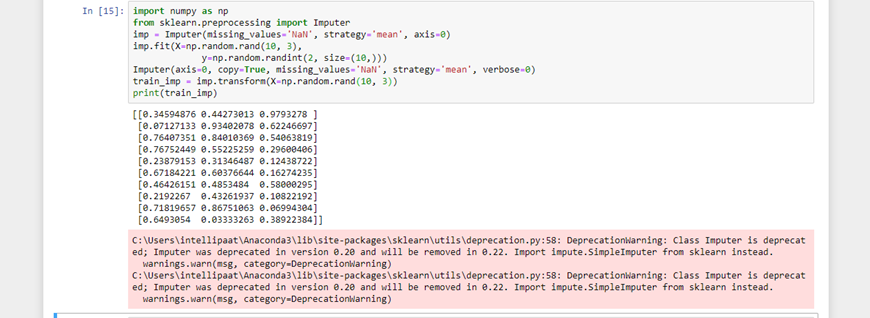# How to get SVMs to play nicely with missing data in scikit-learn?

1 view

I am using scikit-learn for some data analysis, and my dataset has some missing values (represented by NA). I load the data in with genfromtxt with dtype='f8' and go about training my classifier.

The classification is fine on RandomForestClassifier and GradientBoostingClassifier objects, but using SVC from sklearn.svm causes the following error:

```    probas = classifiers[i].fit(train[traincv], target[traincv]).predict_proba(train[testcv])
File "C:\Python27\lib\site-packages\sklearn\svm\base.py", line 409, in predict_proba
X = self._validate_for_predict(X)
File "C:\Python27\lib\site-packages\sklearn\svm\base.py", line 534, in _validate_for_predict
X = atleast2d_or_csr(X, dtype=np.float64, order="C")
File "C:\Python27\lib\site-packages\sklearn\utils\validation.py", line 84, in atleast2d_or_csr
assert_all_finite(X)
File "C:\Python27\lib\site-packages\sklearn\utils\validation.py", line 20, in assert_all_finite
raise ValueError("array contains NaN or infinity")
ValueError: array contains NaN or infinity```

What gives? How can I make the SVM play nicely with the missing data? Keeping in mind that the missing data works fine for random forests and other classifiers..

by (8k points)

You can do data imputation to handle missing values before using SVM.

import numpy as np

from sklearn.preprocessing import Imputer

imp = Imputer(missing_values='NaN', strategy='mean', axis=0)

imp.fit(train)

Imputer(axis=0, copy=True, missing_values='NaN', strategy='mean', verbose=0)

train_imp = imp.transform(train)